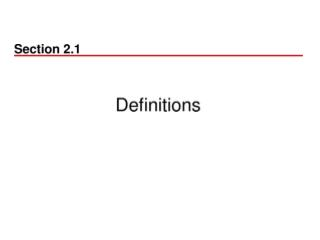DownloadDownload PresentationEX

# EX

Download Presentation## EX

- - - - - - - - - - - - - - - - - - - - - - - - - - - E N D - - - - - - - - - - - - - - - - - - - - - - - - - - -
##### Presentation Transcript

1. EX • A fair coin is tossed 3 times and let X be the random variable of number of heads in the 3 tosses. {X = 0} => {(TTT)} {X = 1} => {(TTH),(THT),(HTT)}

2. EX • P(X = 0) = P{(TTT)} = 1/8 • P(X = 1) = P{(TTH),(THT),(HTT)} = 3/8 • P(X = 2) = P{(THH),(HHT),(HTH)) = 3/8 • P(X=3) = P{(HHH)} = 1/8

3. x 2 3 4 5 6 7 8 9 10 11 12 6/36 5/36 4/36 3/36 2/36 1/36 The PMF of tossing two dies

4. ANS.

5. Ex • Three balls are to be randomly selected (without replacement) from an urn containing 20 balls numbered 1 to 20 • If we bet that at least one of the drawn balls has a number at least 17, what is the probability of winning?

6. Ans. • Let X denote the largest number selected. • P(X >= 17) = P(x=17)+P(x=18)+P(x=19)+P(x=20) • = 16C2 / 20C3+17C2 / 20C3+18C2 / 20C3+19C2 / 20C3

7. Ex • Three balls are randomly chosen from an urn containing 3 white, 3 red, and 5 black balls. Suppose that we win \$1 for each white ball selected and lose \$1 for each red ball selected. The probability that we win money ?

8. Ans • Let X denote our total winning from the experiment. • P(X=1) = ((3C1*5C2)+ (3C2*3C1))/ 11C3 • P(X=2) = (3C2*5C1) / 11C3 • P(X=3) = (3C3) / 11C3

9. Bernoulli random variable • An experiment has two possible outcomes, called “success” and “failure”: sometimes called a Bernoulli trial • The probability of success is p • X = 1 if success occurs, X = 0 if failure occurs Then p(0) = P{X = 0} = 1 – p and p(1) = P{X = 1} = p X is a Bernoulli random variable with parameter p.

10. 負二項分配Negative Binomial Random Variables